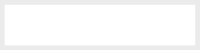# Calculator

Calculator for calculating the weight and length of STEEL ROD with a specific gravity of 7.85 kN/m3

diameter:  mm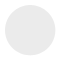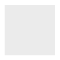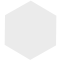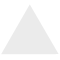Calculator for calculating the weight and length of SHEETS, PLATES AND FLAT BARS with a specific gravity of 7.85 kN/m3

width:  mm

thickness:  mmCalculator for calculating the weight and length of PIPES with a specific gravity of 7.85 kN/m3

width:  mm

thickness:  mm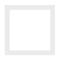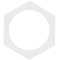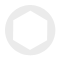Calculator for calculating the weight and length of RECTANGULAR PIPES with a specific gravity of 7.85 kN/m3

width:  mm

height:  mm

thickness:  mm